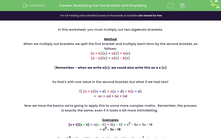# Multiplying Out Two Brackets and Simplifying

In this worksheet, students multiply out two binomials.Key stage:  KS 3

Curriculum topic:   Algebra

Curriculum subtopic:   Simplify Algebraic Expressions to Maintain Equivalence

Difficulty level:#### Worksheet Overview

In this worksheet, you must multiply out two algebraic brackets.

Method

When we multiply out brackets we split the first bracket and multiply each term by the second bracket, as follows:

(a + b)(c) = a(c) + b(c)

(a - b)(c) = a(c) - b(c)

(Remember - when we write a(c), we could also write this as a x (c)

So that's with one value in the second bracket, but what if we had two?

1) (a + b)(c + d) = a(c + d+ b(c + d)

=  ac + ad + bc + bd

Now we have the basics we're going to apply this to some more complex maths.  Remember, the process is exactly the same, even if it looks a bit more intimidating.

Examples

(x + 3)(x - 6) = x(x - 6) + 3(x - 6) = x- 6x + 3x - 18
= x- 3x - 18

(2x - 1)2 = (2x - 1)(2x - 1) = 2x(2x - 1) - 1(2x - 1) = 4x- 2x - 2x + 1
= 4x- 4x + 1

### What is EdPlace?

We're your National Curriculum aligned online education content provider helping each child succeed in English, maths and science from year 1 to GCSE. With an EdPlace account you’ll be able to track and measure progress, helping each child achieve their best. We build confidence and attainment by personalising each child’s learning at a level that suits them.

Get started